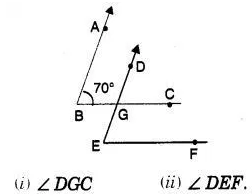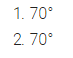# In the given figure, the arms of two angles are parallel. If ∠ ABC = 70°, then find

In the given figure, the arms of two angles are parallel. If ∠ ABC = 70°, then find